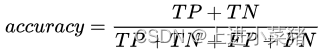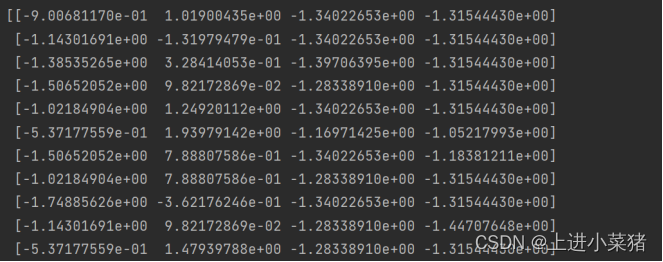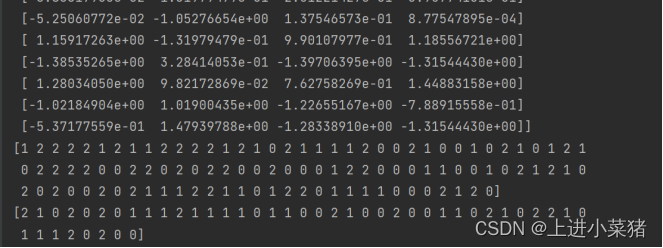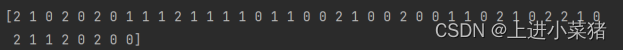# KNN 算法及性能评估之鸢尾花特征分类【机器学习】

• 2022 年 7 月 19 日
• 本文字数：1307 字

阅读完需：约 4 分钟# 一.前言

## 1.1 本文原理

KNN 算法：通过在整个训练集中搜索 k 个最相似的实例（邻居）并汇总这些 k 个实例的输出变量来预测新的数据点。它可以用于分类和回归，是一种监督学习算法。混淆矩阵：至少有 m*m 的表。前 m 行和 m 列的条目 CMJ 表示分类器标记为 J 的类 I 元组数。

## 1.2 本文目的

1. 使用 scikit-learn 的数据归一化函数，对鸢尾花数据进行归一化；

2. 使用 scikit-learn 的切分数据集函数，将鸢尾花数据切分为训练数据集和测试数据集；

3. 使用 scikit-learn 的 KNN 算法，对鸢尾花进行分类训练和测试（即预测）；

4. 使用 scikit-learn 的混淆矩阵函数，显示性能评估的混淆矩阵以及准确率，并分析混淆矩阵的内容；

# 二.实验过程

## 2.1 使用 scikit-learn 的数据归一化函数，对鸢尾花数据进行归一化；

from sklearn.datasets import load_irisiris = load_iris()X = iris.datay = iris.target

from sklearn.metrics import confusion_matrix,accuracy_score,recall_score,precision_scorefrom sklearn.model_selection import train_test_splitfrom sklearn import preprocessingiris_X=preprocessing.scale(X)

print(iris_X)## 2.2 使用 scikit-learn 的切分数据集函数，将鸢尾花数据切分为训练数据集和测试数据集；

X_train,X_test,y_train,y_test =train_test_split(iris_X,y,test_size=0.3,random_state=0)

print(X_train)print(X_test)print(y_train)print(y_test)## 2.3 使用 scikit-learn 的 KNN 算法，对鸢尾花进行分类训练和测试（即预测）；

KNN 分类模型如下：引入 k 近邻算法模块：

from sklearn import neighbors

KNeighborsClassifier 用于实现 k 近邻投票算法的分类器如下：

model=neighbors.KNeighborsClassifier(n_neighbors=3)

model.fit(X_train,y_train)

v_pred=model.predict(X_test)

print(v_pred)## 2.4 使用 scikit-learn 的混淆矩阵函数，显示性能评估的混淆矩阵以及准确率，并分析混淆矩阵的内容；

confusion_matrix(y_test,v_pred)

print(confusion_matrix(y_test,v_pred))print("准确率：%.3f"% accuracy_score(y_test,v_pred))## 2.5 分析混淆矩阵的内容以及总结

sklearn.metrics.confusion_matrix(y_true, y_pred, labels=None, sample_weight=None)y_true: 是样本真实分类结果 y_pred: 是样本预测分类结果 labels：是所给出的类别，通过这个可对类别进行选择 sample_weight : 样本权重预测正确的结果占总样本的百分比的 97.8，本次 KNN 算法对鸢尾花进行分类训练和测试效果非常的准确。总结：1.熟悉机器学习之 KNN 算法及性能评估方法 2.使用 KNN 算法解决问题并做性能评估## 评论